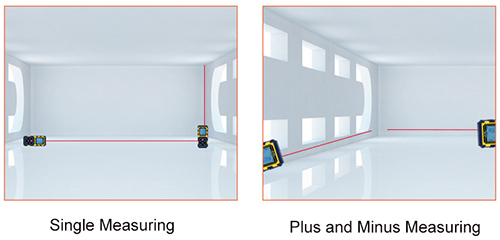All Categories

# How does Laser Distance Meter Work?

1. The laser distance meter uses the principle of infrared distance measurement or laser distance measurement. The principle of laser ranging can basically be ascribed to measure the time required for the light to go back and forth to the target, and then the distance D is calculated by the speed of light c=299792458m/s and the atmospheric refraction coefficient n. Because it is difficult to measure time directly, it is usually to measure the phase of continuous wave, which is called phase measuring distance meter. Of course, there are also pulsed distance measures. It should be noted that phase measurement is not to measure the phase of infrared or laser, but to measure the phase of the signal modulated on infrared or laser. The hand-held laser distance meter used for house surveying in the construction industry, applies the same principle.

2. The plane of the measured object must be perpendicular to the light. Usually precision ranging requires the cooperation of total reflection prisms, and the distance meter used for house measurement directly uses smooth wall reflection to measure, mainly because the distance is relatively short, and the signal intensity of the light reflection is large enough. It can be seen that the plane of the object to be measured must be perpendicular to the light; otherwise if the return signal is too weak, the precise distance cannot be measured.3. The plane of the measured object is diffuse reflection. In actual engineering, a thin plastic plate will be used as a reflecting surface to solve the serious problem of diffuse reflection.

In optical distance measurement, laser distance finders are often used as they are featured with characteristics of monochromaticity, good coherence, and strong directionality for high-precision measurement and detection, such as measuring length, distance, speed, angle, and so on. Laser ranging can be divided into pulsed laser ranging and continuous wave phase laser ranging in the ways of technology.

Entertainment level pulse laser distance meter products can achieve display accuracy of 1m and measurement accuracy of ±1m. Measurement-level pulsed laser distance meter products display accuracy of 0.1m and measurement accuracy of ±0.15m. The phase laser rangefinder can reach accuracy of 1mm error, which is suitable for various high-precision measurement purposes.

The principle of pulsed laser ranging is similar to radar ranging: The laser distance meter emits a laser signal to the target, and it will be reflected back when it hits the target. Since the propagation speed of light is known, so we only need to record the round-trip time of the optical signal, then compute the measured distance by the speed of light (300,000 km/s) being multiplied by one-half of the round-trip time. The hand-held distance measures, which are widely used now, have a range of hundreds of meters to tens of kilometers for operation distance, with measurement accuracy of about five meters. The high-precision distance meter, which is developed by China for measuring the range of satellites, has a measurement accuracy of several centimeters.Continuous-wave phase laser ranging is to irradiate the measured target with a continuously modulated laser beam, and then convert to the distance of the measured target by measuring the phase change caused by the beam to and fro. In order to ensure measurement accuracy, a laser reflector is generally installed on the target. The relative error measured by the phase laser rangefinder is one in million.

In addition, the laser distance meter can be also combined with microwave radar, to take advantage of the narrow laser wave speed and make up for the lack of interference from the ground when microwave radar is working at low elevation angles. It can also be combined with optical theodolite, infrared and TV tracking systems to form a photoelectric tracking measurement system, which can be used as a measuring device for range testing and photoelectric fire control system for weapons. This kind of laser distance meter has been widely used in the fire control system of ground artillery and tank guns, which improve the hit rate greatly.

Tool.com provides the best price 40m laser distance meter/ 60m laser distance meter, etc. Contact us to buy now.